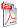Issue archive

https://doi.org/10.15255/KUI.2019.010
Published: Kem. Ind. 68 (11-12) (2019) 573–582
Paper reference number: KUI-10/2019
Paper type: Original scientific paper
Download paper:PDFComparative Study of Predicting the Molecular Diffusion Coefficient for Polar and Non-polar Binary Gas Using Neural Networks and Multiple Linear Regressions

N. Melzi, L. Khaouane, Y. Ammi, S. Hanini, M. Laidi and H. Zentou

##### Abstract

In the current study, an artificial neural network (ANN) and multiple linear regressions (MLR) have been used to develop predictive models for the estimation of molecular diffusion coefficients of 1252 polar and non-polar binary gases at multiple pressures over a wide range of temperatures and substances. The quality and reliability of each method were estimated in terms of the correlation coefficient (R), mean squared errors (MSE), root mean squared error (RMSE), and in terms of external validation coefficients (Q2ext). The comparison between the artificial neural network (ANN) and the multiple linear regressions (MLR) revealed that the neural network models showed a good predicting ability with lower errors (the roots of the mean squared errors in the total database were 0.1400 for ANN1 and 0.1300 for ANN2), and (root mean squared errors in the total databases were 0.5172 for MLR1 and 0.5000 for MLR2).

##### Keywords

prediction, molecular diffusion, neural networks, multiple linear regressions